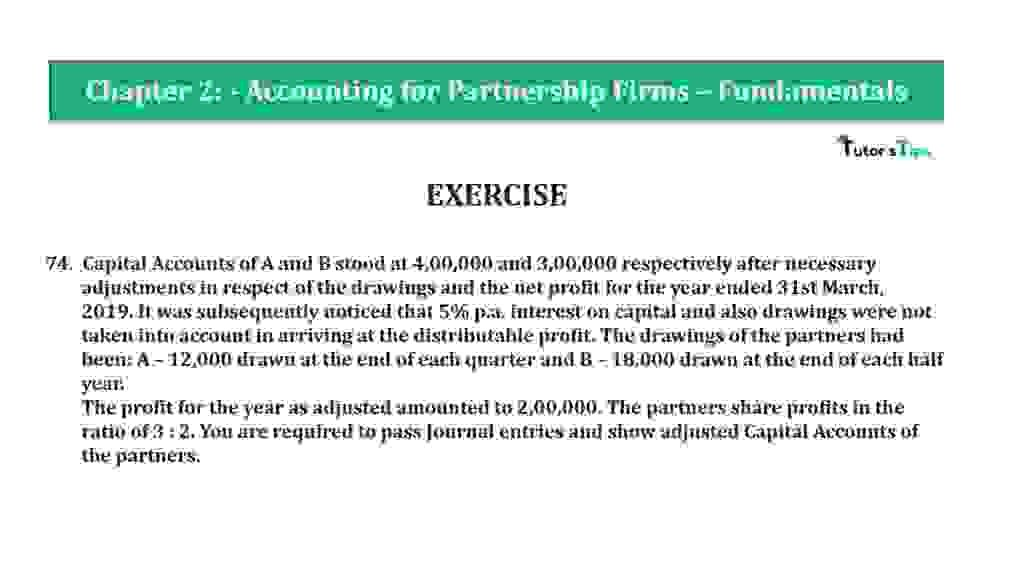# Question 74 Chapter 2 of +2-A – T.S. Grewal 12 Class Part – A Vol. 1Question 74 Chapter 2 of +2-A

74. Capital Accounts of A and B stood at 4,00,000 and 3,00,000 respectively after necessary adjustments in respect of the drawings and the net profit for the year ended 31st March 2019. It was subsequently noticed that 5% p.a. interest on capital and also drawings were not taken into account in arriving at the distributable profit. The drawings of the partners had
been: A – 12,000 drawn at the end of each quarter and B – 18,000 drawn at the end of each half-year.
The profit for the year as adjusted amounted to 2,00,000. The partners share profits in the ratio of 3: 2. You are required to pass Journal entries and show adjusted Capital Accounts of
the partners.

The solution of Question 74 Chapter 2 of +2-A

:

 Date Particulars L.F. Debit Credit A’s Capital A/c Dr 1,210 To B’s Capital A/c 1,210 (Being adjustment made)

 Particulars A B Particulars A B To B’s Capital A/c 1,210 – By Balance B/d 4,00,000 3,00,000 By A’s Capital A/c *1 – 1,210 To Balance c/d 3,98,790 3,01,210 4,00,000 3,01,210 4,00,000 3,01,210

Working Note: –

 Statement Showing Adjustment of Profit required Particulars Amount of A’s Capital Amount of B’s Capital Capital at the end 4,00,000 3,00,000 Less: Profit alreadyDistributed (3:2) 1,20,000 80,000 Add: Drawings During the year 48,000 36,000 Capital at the beginning 3,28,000 2,56,000

 Statement Showing Adjustment of Profit required Particulars A B Total Amount of Interest on Capital @5% p.a.*2 16,400 12,800 29,200 Less: Amount of Interest on Drawing @5% p.a.*3 & *4 – 900 – 450 – 1,350 Actual total amount due – will be distributed as 15,500 12,350 27,850 Less: – Wrongly total amount distributed in the profit sharing ration i.e. 3: 2 – 16,710 – 11,140 22,760 – 1,210 1,210 – A get extra so we have to debit his capital a/c with difference amount B get less amount, so we have to credit his capital a/c with difference amount

*2 Calculation of Actual Amount of Interest on A’s, B’s, Capital
Interest on Capital = Opening Capital X Rate of Interest

 Interest on A’s Capital = 3,28,000 X 5 100

Interest on A’s Capital = 16,400/-

 Interest on B’s Capital = 2,56,000 X 5 100

Interest on B’s Capital = 12,800/-

*3 Calculation of interest on A’s Drawings
If partners withdrawal the same amount of drawing on a regular basis then we can calculate the interest on drawing with help of following formula: –

Interest on Drawing = Total Drawing X Rate of Interest X Period/12 Months
Rate of Interest = 5%
Period = the period/time will be calculated with the help of following formula: –

 Period = Time left after 1st withdrawal + Time left after Last withdrawal 2

Withdrawal at the end of each quarter = 12,000
It means 1st withdrawal has made on 30/06/2019
So, Time left from 30/06/2019 to 31/03/2020
are 9 Months
And last withdrawal has made on 31/03/2020
So, Time left from 31/03/2020 to 31/03/2020
are 0 Month and 0 day
Now, put this time period in the formula

 Period = 9 + 0 2

 Period = 9 2

Period = 4.5

 total Drawing = 12,000 * 4 = 48,000 Multiplied by 4 because a number of the quarter in the year is 4.
 Interest on Drawing = 48,000 X 5 X 4.5 100 12

Interest on Drawing = 900/-

*4 Calculation of interest on B’s Drawings
If partners withdrawal the same amount of drawing on a regular basis then we can calculate the interest on drawing with help of following formula: –
Interest on Drawing = Total Drawing X Rate of Interest X Period/12 Months
Rate of Interest = 5%
Period = the period/time will be calculated with the help of following formula: –

 Period = Time left after 1st withdrawal + Time left after Last withdrawal 2

B drew at the end of each half-year = 18,000
It means 1st withdrawal has made on 30/09/2019
So, Time left from 30/09/2019 to 31/03/2020
are 6 Months
And last withdrawal has made on 31/03/2020
So, Time left from 31/03/2020 to 31/03/2020
are 0 Month and 0 day

Now, put this time period in the formula

 Period = 6 + 0 2

Also, Check out the solved question of previous Chapters: –

### T.S. Grewal’s Double Entry Book Keeping +2 (Vol. I: Accounting for Not-for-Profit Organizations and Partnership Firms)

• Chapter No. 1 – Financial Statement of Not-For-Profit Organisations
• Chapter No. 2 – Accounting for Partnership Firms – Fundamentals
• Chapter No. 3 – Goodwill: Nature and Valuation
• Chapter No. 4 – Change in Profit-Sharing Ratio Among the Existing Partners
• Chapter No. 5 – Admission of a Partner
• Chapter No. 6 – Retirement/Death of a Partner
• Chapter No. 7 – Dissolution of a Partnership Firm

### T.S. Grewal’s Double Entry Book Keeping (Vol. II: Accounting for Companies)

• Chapter No. 1 – Financial Statements of a Company
• Chapter No. 2 – Financial Statement Analysis
• Chapter No. 3 – Tools of Financial Statement Analysis – Comparative Statements and Common- Size Statements
• Chapter No. 4 – Accounting Ratios
• Chapter No. 5 – Cash Flow StatementVol. I: Accounting for Not-for-Profit Organizations and Partnership Firms